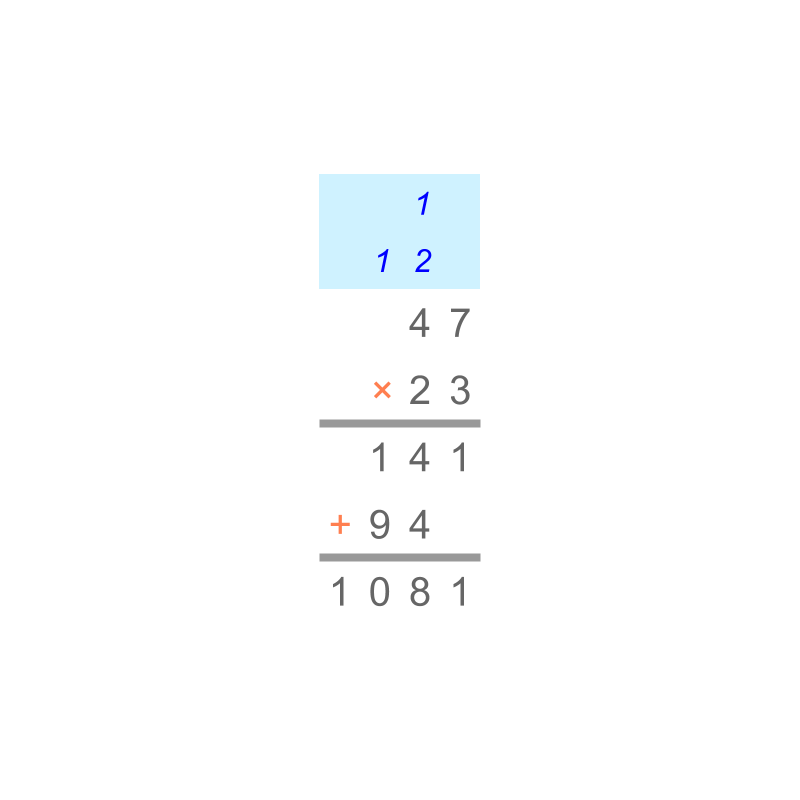maths > decimals

Decimal Multiplication

what you'll learn...

overview

Decimals are fractions with standard place values. In this page, the following for decimals are explained.

•  multiplication in first principles -- repeatedly combining a quantity and measuring the combined and

•  simplified procedure : Sign property of multiplication and Multiplication by Place value for Decimals.

Multiplication can be understood as "multiplicand repeated multiplier number of times".

Decimals are fractions with standardized place-values.

Consider $0.2×0.3$$0.2 \times 0.3$.

By first principles, the multiplicand $0.2=\frac{2}{10}$$0.2 = \frac{2}{10}$ is repeated multiplier $0.3=\frac{3}{10}$$0.3 = \frac{3}{10}$ number of times.

This is done in two steps,

first step: $\frac{2}{10}$$\frac{2}{10}$ repeated $\frac{1}{10}$$\frac{1}{10}$ (denominator of $\frac{3}{10}$$\frac{3}{10}$) times is $\frac{2}{100}$$\frac{2}{100}$.

Second step: the result from the first step $\frac{2}{100}$$\frac{2}{100}$ is repeated $3$$3$ (numerator of $\frac{3}{10}$$\frac{3}{10}$) times is $\frac{2}{100}+\frac{2}{100}+\frac{2}{100}$$\frac{2}{100} + \frac{2}{100} + \frac{2}{100}$ $=\frac{6}{100}$$= \frac{6}{100}$

The product is $\frac{6}{100}$$\frac{6}{100}$, or equals $0.06$$0.06$ in decimals.

aligned or opposed to direction

Decimals are directed numbers, that is decimals are either positive or negative.

$0.2$$0.2$ aligned in direction is "$+0.2$$+ 0.2$".

$0.2$$0.2$ opposed in direction is "$-0.2$$- 0.2$".

Directed numbers, positive and negative, are explained as "aligned in direction" and "opposed in direction" respectively.

Consider $0.2×\left(-0.3\right)$$0.2 \times \left(- 0.3\right)$.

By first principles, the multiplier $0.2=\frac{2}{10}$$0.2 = \frac{2}{10}$ aligned in direction is repeated multiplier $0.3=\frac{3}{10}$$0.3 = \frac{3}{10}$ opposed in direction number of times.

This is done in two steps,

first step: $\frac{2}{10}$$\frac{2}{10}$ repeated $\frac{1}{10}$$\frac{1}{10}$ (denominator of $\frac{3}{10}$$\frac{3}{10}$) times is $\frac{2}{100}$$\frac{2}{100}$.

Second step: the result from the first step $\frac{2}{100}$$\frac{2}{100}$ is repeated $3$$3$ (numerator of $\frac{3}{10}$$\frac{3}{10}$) timesopposed in direction is $\frac{2}{100}+\frac{2}{100}+\frac{2}{100}$$\frac{2}{100} + \frac{2}{100} + \frac{2}{100}$ $=\frac{6}{100}$$= \frac{6}{100}$ opposed in direction.

The product is $\frac{6}{100}$$\frac{6}{100}$ opposed in direction, or equals $-0.06$$- 0.06$ in decimals.

Decimal multiplication by first principles : Decimal multiplication is repeating the multiplicand, multiplier number of times with sign of the numbers (direction) handled appropriately.

simplifications recapIn whole numbers, we have studied Multiplication by Place-value as illustrated in the figure. This procedure is used in decimals in a later step.

In Integers, we have studied Sign-property of Multiplication.
+ve $×$$\times$ +ve = +ve
+ve $×$$\times$ -ve = -ve
-ve $×$$\times$ +ve = -ve
-ve $×$$\times$ -ve = +ve
This is applicable to decimals.

In Fractions, we have studied Multiplication of Numerators and Denominators.
For example, to multiply $\frac{4}{5}×\frac{3}{2}$$\frac{4}{5} \times \frac{3}{2}$, the numerators are multiplied and denominators are multiplied. The product is $\frac{4×3}{5×2}$$\frac{4 \times 3}{5 \times 2}$.

Similarly decimals are multiplied keeping in mind the place-value representation.
For example, to multiply $0.8×1.5$$0.8 \times 1.5$, it is equivalently thought as $\frac{8}{10}×\frac{15}{10}$$\frac{8}{10} \times \frac{15}{10}$ and so the product is $\frac{8×15}{10×10}$$\frac{8 \times 15}{10 \times 10}$. Note that $8×15$$8 \times 15$ is multiplied as per "multiplication by place-value".

simplify decimal multiplication

Consider multiplication of $0.007×0.05$$0.007 \times 0.05$
This is equivalently $\frac{7}{1000}×\frac{5}{100}$$\frac{7}{1000} \times \frac{5}{100}$
$=\frac{7×5}{1000×100}$$= \frac{7 \times 5}{1000 \times 100}$

Understanding the above, a simplified procedure to multiply the decimals is devised.

The decimal point of multiplicand and multiplier are removed and the numbers are multiplied as integers.
eg: $0.007$$0.007$ is modified to the integer form $7$$7$.
$0.05$$0.05$ is modified to the integer form $5$$5$.

The number of decimal-places in the multiplicand and multiplier are counted.
eg: $0.007$$0.007$ has $3$$3$ decimal-places.
$0.05$$0.05$ has $2$$2$ decimal-places.

Now the integer forms are multiplied.
eg: $7×5=35$$7 \times 5 = 35$

The number of decimal places of multiplicand and multiplier are added.
eg: $3+2=5$$3 + 2 = 5$.

The product of integer forms is modified to have the number of decimal points give by the sum above.
eg: $35$$35$ is modified to $0.00035$$0.00035$ which has $5$$5$ decimal points.

example

What is $12.55×0.002$$12.55 \times 0.002$
The answer is "$0.02510$$0.02510$".
$1255×2=2510.$$1255 \times 2 = 2510.$

Total number of decimal places in the multiplicand and multiplier is $2+3=5$$2 + 3 = 5$
So the product decimal place moves $5$$5$ places

$12.55×0.002=0.02510$$12.55 \times 0.002 = 0.02510$

summary

Decimal Multiplication -- Simplified Procedure : The signs (+ve / -ve) are handled as in Sign-property of Integer Multiplication
•  +ve $×$$\times$ +ve = +ve
•  +ve $×$$\times$ -ve = -ve
•  -ve $×$$\times$ +ve = -ve
•  -ve $×$$\times$ -ve = +ve

The decimal places are removed and the multiplication is carried out as per Whole number Multiplication by Place Value.
•  The decimal place is re-introduced in to the product.
•  The decimal-point is moved to the left -- a number of digits equal to the total number of decimals in multiplier and multiplicand

Outline